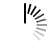# How to round off a floating number using Python?

How to round off a floating number using Python?

The round() function in Python's library rounds the number to given position. Following are some examples.

```>>> round(11.6912,2) # upto second decimal place
11.69
>>> round(11.6912,1) # upto first place after decimal point
11.7
>>> round(11.6912) # rounded to nearest integer
12
>>> round(11.6912,-1)#rounded to ten's place
10.0```